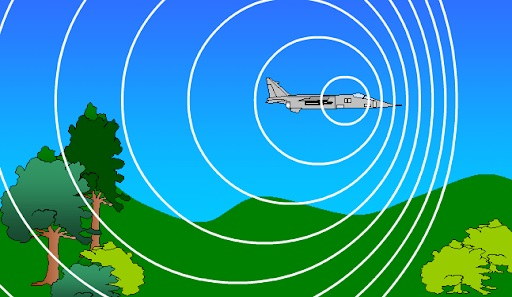# Application of Doppler EffectDoppler effect is defined as the change in the frequency and wavelength of the wave. Doppler effect takes place when there is a change in the distance between the creator of the wave and the observer.

Use in Flow Measurement

Doppler effect is used in flow measurement. Reflected ultrasonic sound is used for measuring the fluid velocity. The relative motion can be measured by measuring the change in the frequency between ultrasonic frequency source, receiver, and the fluid carrier.

Following is the formula used for calculating the fluid velocity:

 v = [c(fr -ft)/(2ft cosФ)]

Where,

• fr is the received frequency
• ft is the transmission frequency
• v is the fluid flow velocity
• Ф is the relative angle between the ultrasonic frequency and the fluid flow

Doppler effect use in flow measurement has advantages, such as it is corrosion-resistant, can be installed in pipes, and the power consumption is relatively low.

This also has a few limitations such as the performance of the flow meter is dependent on the flow profile, particle density, and temperature.

Doppler Echocardiogram

In a conventional echocardiogram, sound waves are used for obtaining the images of the heart. A transducer is used for transmitting and receiving ultrasound waves, which gets reflected when the waves reach densities that are different from each other. An echocardiogram is used for understanding the heart structures but the flow of blood cannot be studied. Doppler effect helps in understanding the flow of blood. The frequency shift in the heart can be studied when the sound waves bounce from blood cells. This change in frequency is used by cardiologists to determine the speed and direction of blood flow.

Doppler effect in Astronomy

Doppler effect can be observed in sound waves just like light waves. Astronomers use the Doppler effect to determine the speed of stars and galaxies. There are two terms that are used for explaining the Doppler effect in astronomy and they are Redshift: It is defined as the displacement of the spectral lines, such that there is an increase in the wavelength. It is used for understanding the stretch in the wavelength of the light.

Blueshift: This phenomenon is the opposite of redshift, that is the spectral lines decrease in wavelength.

Interested to learn more about the other concepts of Physics such as stress-strain curve, stay tuned with BYJU’S.